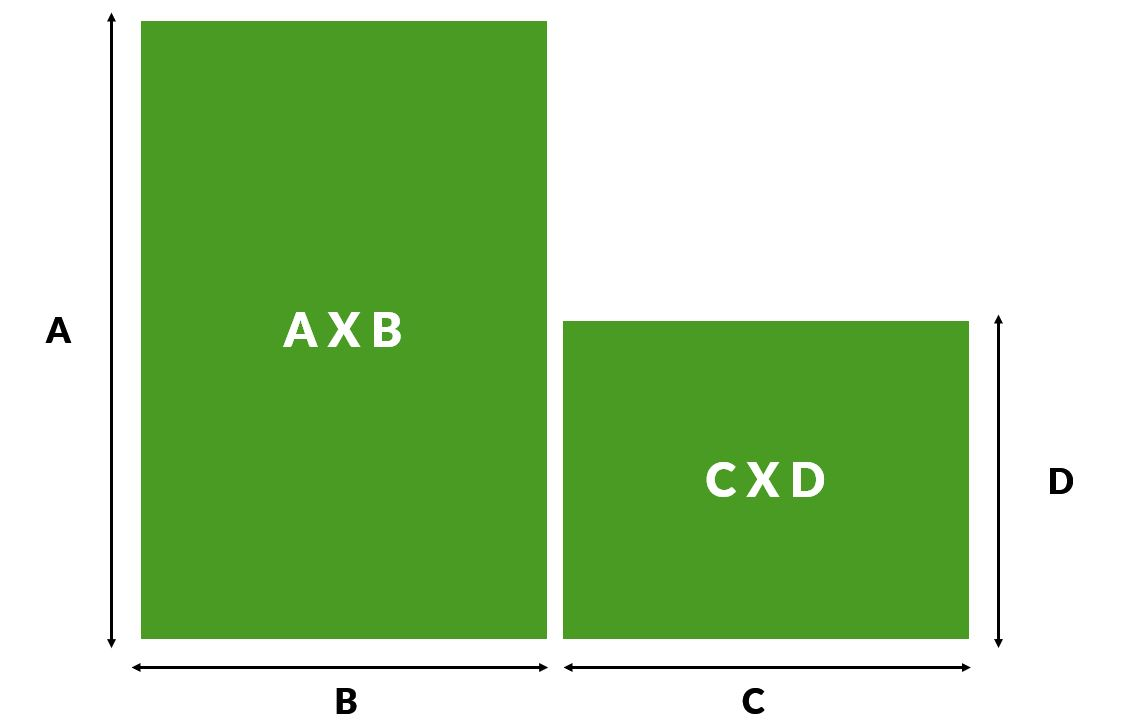# How Much Artificial Grass Do I Need?

One of the first steps in planning for an artificial grass installation is to calculate how much of it you will need to fill the desired area. We understand that gardens, parks and fields come in all different shapes and sizes, so in this guide, we will provide instructions on how to measure different shapes in order to calculate how much artificial grass you will need.

To measure rectangular and square-shaped gardens, simply multiply the width by the length, which will give you the number of square metres of artificial grass you need. As an example, if the length was 5 metres and the width was 6 metres, this area would require 30 square metres of grass.

If your lawn has an angled or shaped section, we recommend the method of using a single section of grass in that area to calculate how much grass you would need. For circular or oval areas of grass, measure the length of the two widest sections and multiply them together.To calculate the area of an L shaped garden, much like with an L shaped roof, the easiest method is to divide the area up into two sections as shown in the diagram below; you can then calculate their areas individually and add them together to get the total number of square metres of artificial grass needed.When it comes to purchasing, we offer artificial grass in 25m x 4m rolls as well as the option to buy by the metre in 1m increments (all on a 4m roll).

In simplest terms, if you divide the square metre total that your installation requires by four (the width of our rolls), the number you get is the number of metres you will need in length. As an example, if the square metre total of the area you want to turf is 24 metres, you will need a six-metre length roll of artificial grass.